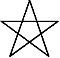Art, History, Music, Politics, and Spirituality For The Modern Alchemist – circulation in over 129 countries

# The Sign of Nudzuchi Year 18,002 Begins: February 8th 2015The Sign of Nudzuchi Year 18,002 Begins: February 8th 2015

There are eight months in the Calendar of Mu, sometimes referred to as the Nyarzirian Calendar. Each month consists of forty-five days, and represents a system of the eight hexagrams moving through the five elements. The Ninzuwu Priest/Priestess invokes the mudra/mantra formula associated with each hexagram that rules a particular day after invoking the Armor of Amaterasu Ohkami. The chart below illustrates the daily Vasuh letters invoked and the ruling “deity” for the said hexagram:
1. February 8th 2015 = Orogorojima = 2nd Hexagram = Shki-Lewhu-Zhee
2. February 9th 2015 = Ahaji = 7th Hexagram = Zhee-Shki-Hmu-Nzu
3. February 10th 2015 = Iyonofutana = 11th Hexagram = Tuu-Aum-Nzu
4. February 11th 2015 = Oki = 15th Hexagram = Zhee-Bnhu
5. February 12th 2015 = Tsukushi = 19th Hexagram = Phe-Lewhu
6. February 13th 2015 = Sado = 24th Hexagram = Zhee-Shki-Tuu-Tuu
7. February 14th 2015 = Tsushima = 36th Hexagram = Zhee-Lewhu-Zhee-Bnhu
8. February 15th 2015 = Shinatohe = 46th Hexagram = Aum-Zhee-Phe-Tuu
9. February 16th 2015 == Entering Earth Element
10. February 17th 2015 = Orogorojima = 2nd Hexagram = Shki-Lewhu-Zhee
11. February 18th 2015 = Ahaji = 7th Hexagram = Zhee-Shki-Hmu-Nzu
12. February 19th 2015 = Iyonofutana = 11th Hexagram = Tuu-Aum-Nzu
13. February 20th 2015 = Oki = 15th Hexagram = Zhee-Bnhu
14. February 21st 2015 = Tsukushi = 19th Hexagram = Phe-Lewhu
15. February 22nd 2015 = Sado = 24th Hexagram = Zhee-Shki-Tuu-Tuu
16. February 23rd 2015 = Tsushima = 36th Hexagram = Zhee-Lewhu-Zhee-Bnhu
17. February 24th 2015 = Shinatohe = 46th Hexagram = Aum-Zhee-Phe-Tuu
18. February 25th 2015 == Entering Water Element
19. February 26th 2015 = Orogorojima = 2nd Hexagram = Shki-Lewhu-Zhee
20. February 27th 2015 = Ahaji = 7th Hexagram = Zhee-Shki-Hmu-Nzu
21. February 28th 2015 = Iyonofutana = 11th Hexagram = Tuu-Aum-Nzu
22. March 1st 2015 = Oki = 15th Hexagram = Zhee-Bnhu
23. March 2nd 2015 = Tsukushi = 19th Hexagram = Phe-Lewhu
24. March 3rd 2015 = Sado = 24th Hexagram = Zhee-Shki-Tuu-Tuu
25. March 4th 2015 = Tsushima = 36th Hexagram = Zhee-Lewhu-Zhee-Bnhu
26. March 5th 2015 = Shinatohe = 46th Hexagram = Aum-Zhee-Phe-Tuu
27. March 6th 2015 == Entering Fire Element
28. March 7th 2015 = Orogorojima = 2nd Hexagram = Shki-Lewhu-Zhee
29. March 8th 2015 = Ahaji = 7th Hexagram = Zhee-Shki-Hmu-Nzu
30. March 9th 2015 = Iyonofutana = 11th Hexagram = Tuu-Aum-Nzu
31. March 10th 2015 = Oki = 15th Hexagram = Zhee-Bnhu
32. March 11th 2015 = Tsukushi = 19th Hexagram = Phe-Lewhu
33. March 12th 2015 = Sado = 24th Hexagram = Zhee-Shki-Tuu-Tuu
34. March 13th 2015 = Tsushima = 36th Hexagram = Zhee-Lewhu-Zhee-Bnhu
35. March 14th 2015 = Shinatohe = 46th Hexagram = Aum-Zhee-Phe-Tuu
36. March 15th 2015 == Entering Air Element
37. March 16th 2015 = Orogorojima = 2nd Hexagram = Shki-Lewhu-Zhee
38. March 17th 2015 = Ahaji = 7th Hexagram = Zhee-Shki-Hmu-Nzu
39. March 18th 2015 = Iyonofutana = 11th Hexagram = Tuu-Aum-Nzu
40. March 19th 2015 = Oki = 15th Hexagram = Zhee-Bnhu
41. March 20th 2015 = Tsukushi = 19th Hexagram = Phe-Lewhu
42. March 21st 2015 = Sado = 24th Hexagram = Zhee-Shki-Tuu-Tuu
43. March 22nd 2015 = Tsushima = 36th Hexagram = Zhee-Lewhu-Zhee-Bnhu
44. March 23rd 2015 = Shinatohe = 46th Hexagram = Aum-Zhee-Phe-Tuu
45. March 24th 2015 == Entering Void Element

#### You may have missed#### One Breath Away by Radio Drive – An Astonishing EP#### The Blackbird Sessions by Sugar Lime Blue ( Album Review)